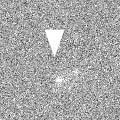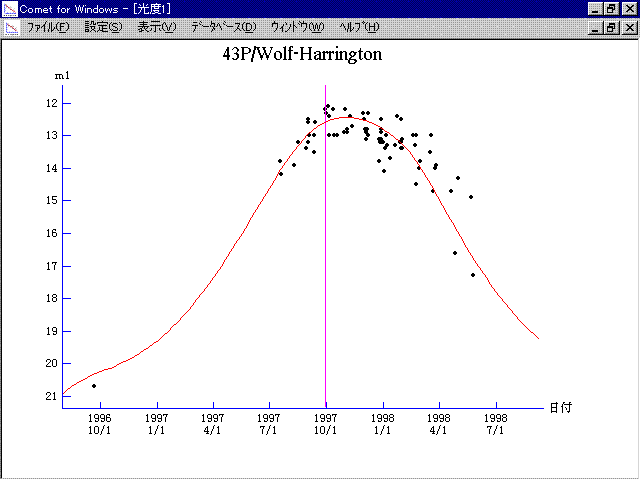# \$B%t%)%k%U(B-\$B%O%j%s%H%sWB@1(B

43P/Wolf-Harrington (1997)###\$B###\$B50F;MWAG(B

```   The following improved orbital elements by Kenji Muraoka,
are from 185 observations 1984 to 1998, including 9 Planets,
Moon and 5 minor planets perturbations and non-gravitational
effect of style II.  The mean residual is +/- 0.72 arc seconds.

Epoch  =  1997 Sept. 29.0  TT      JDT = 2450720.5
T  =  1997 Sept. 29.21665      +/- 0.00080 (m.e.) TT
Peri. =  187.13292                +/- 0.00025
Node  =  254.75600                +/- 0.00007   (2000.0)
Incl. =   18.51038                +/- 0.00002
q  =    1.5818264              +/- 0.0000007 AU
e  =    0.5439775              +/- 0.0000002
a  =    3.4687466              +/- 0.0000006 AU
n  =    0.15256159             +/- 0.00000004
P  =    6.460                  +/- 0.0000017  years
A1  =   +0.369                  +/- 0.017
A2  =   -0.03777                +/- 0.00054
```

###\$B@1?^(B1996\$BG/(B 7\$B7n(B 6\$BF|!A(B1997\$BG/(B 8\$B7n(B10\$BF|(B1997\$BG/(B 8\$B7n(B10\$BF|!A(B1998\$BG/(B12\$B7n(B 3\$BF|(B

###\$B8wEYJQ2=(B

```        m1 = 7.5 + 5 log\$B&\$(B + 20.0 log r  [ ,0]  (              \$B!A(B1997\$BG/(B 9\$B7n(B29\$BF|(B)
m1 = 8.5 + 5 log\$B&\$(B + 15.0 log r  [0, ]  (1997\$BG/(B 9\$B7n(B29\$BF|!A(B              )
```##### \$B50F;MWAG\$OB<2,7r<#;a\$N7W;;\$K\$h\$k\$b\$N\$G\$9!#(B \$B@1?^\$O(B StellaNavigator Ver.2.0 for Windows (\$B%"%9%H%m%"!<%D(B \$BJTCx(B / \$B%"%9%-!<=PHG6I4)(B) \$B\$G:n@.\$7\$?\$b\$N\$G\$9!#(B \$B8wEY%0%i%U\$O(BComet for Windows\$B\$G:n@.\$7\$?\$b\$N\$G\$9!#(B